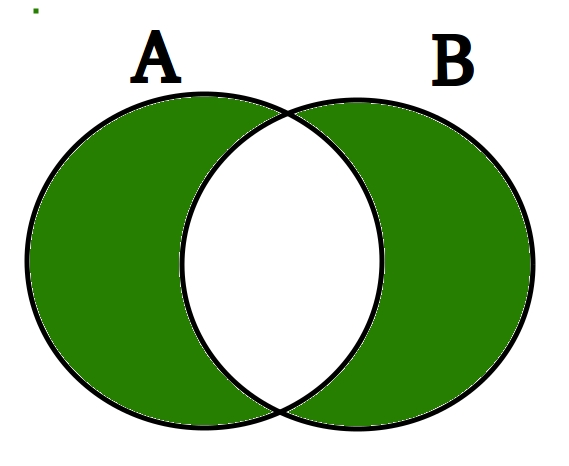Open in App
Not now

# Python Set | symmetric_difference()

• Last Updated : 14 Sep, 2022

Python Set symmetric_difference() Method is used to get the elements present in either of the two sets, but not common to both the sets. Let’s look at the Venn diagram of the symmetric_difference between two sets.Python Set symmetric_difference() Venn diagram

Symmetric Difference is marked in Green If there are a set_A and set_B, then the symmetric difference between them will be equal to the union of set_A and set_B without the intersection between the two.

## Python set symmetric_difference() Method Syntax

Syntax: set_A.symmetric_difference(set_B)

Parameter: Takes a single parameter that has to be a set

Return: Returns a new set which is the symmetric difference between the two sets.

## Python3

 `set_A ``=` `{``1``, ``2``, ``3``, ``4``, ``5``}``set_B ``=` `{``6``, ``7``, ``3``, ``9``, ``4``}``print``(set_A.symmetric_difference(set_B))`

Output:

`{1, 2, 5, 6, 7, 9}`

### Example 1: Finding Symmetric difference using ‘^’ Operator in Python

We can also find symmetric difference from two sets using ‘^’ operator in Python.

## Python3

 `set_A ``=` `{``"ram"``, ``"rahim"``, ``"ajay"``, ``"rishav"``, ``"aakash"``}``set_B ``=` `{``"aakash"``, ``"ajay"``, ``"shyam"``, ``"ram"``, ``"ravi"``}``print``(set_A ^ set_B)`

Output:

`{'shyam', 'ravi', 'rahim', 'rishav'}`

## Python

 `A ``=` `{``'p'``, ``'a'``, ``'w'``, ``'a'``, ``'n'``}``B ``=` `{``'r'``, ``'a'``, ``'o'``, ``'n'``, ``'e'``}``C ``=` `{}` `print``(A.symmetric_difference(B))``print``(B.symmetric_difference(A))` `print``(A.symmetric_difference(C))``print``(B.symmetric_difference(C))`

Output:

```set(['e', 'o', 'p', 'r', 'w'])
set(['e', 'o', 'p', 'r', 'w'])
set(['a', 'p', 'w', 'n'])
set(['a', 'r', 'e', 'o', 'n'])```

My Personal Notes arrow_drop_up